Next: Route to Chaos Up: The Chaotic Pendulum Previous: Basins of Attraction

Period-Doubling Bifurcations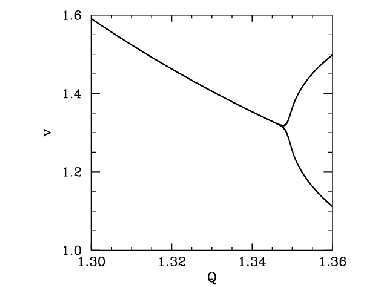Let us now return to Figure 64. Recall, that as the quality-factor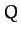is gradually increased, the time-asymptotic orbit of the pendulum through phase-space undergoes a sudden transition, at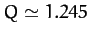, from a left-right symmetric period-1 orbit to a left-favouring period-1 orbit. What happens if we continue to increase? Figure 73 is basically a continuation of Figure 64. It can be seen that asis increased the left-favouring period-1 orbit gradually evolves until a critical value of, which is about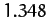, is reached. Whenexceeds this critical value the nature of the orbit undergoes another sudden change: this time from a left-favouring period-1 orbit to a left-favouring period-2 orbit. Obviously, the change is indicated by the forking of the curve in Figure 73. This type of transition is termed a period-doubling bifurcation, since it involves a sudden doubling of the repetition period of the pendulum's time-asymptotic motion.

We can represent period-1 motion schematically as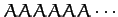, whererepresents a pattern of motion which is repeated every period of the external drive. Likewise, we can represent period-2 motion as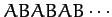, whereandrepresent distinguishable patterns of motion which are repeated every alternate period of the external drive. A period-doubling bifurcation is represented: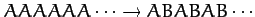. Clearly, all that happens in such a bifurcation is that the pendulum suddenly decides to do something slightly different in alternate periods of the external drive.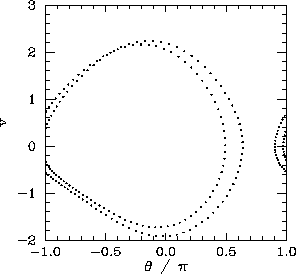Figure 74 shows the time-asymptotic phase-space orbit of the pendulum calculated for a value ofsomewhat higher than that required to trigger the above mentioned period-doubling bifurcation. It can be seen that the orbit is left-favouring (i.e., it spends the majority of its time on the left-hand side of the plot), and takes the form of a closed curve consisting of two interlocked loops in phase-space. Recall that for period-1 orbits there was only a single closed loop in phase-space. Figure 75 shows the Poincaré section of the orbit shown in Figure 74. The fact that the section consists of two points confirms that the orbit does indeed correspond to period-2 motion.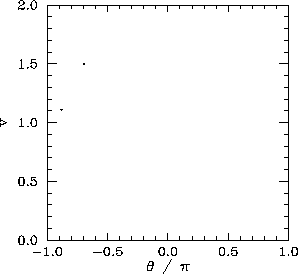A period-doubling bifurcation is an example of temporal symmetry breaking. The equations of motion of the pendulum are invariant under the transformation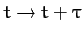, where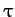is the period of the external drive. In the low amplitude (i.e., linear) limit, the time-asymptotic motion of the pendulum always respects this symmetry. However, as we have just seen, in the non-linear regime it is possible to obtain solutions which spontaneously break this symmetry. Obviously, motion which repeats itself every two periods of the external drive is not as temporally symmetric as motion which repeats every period of the drive.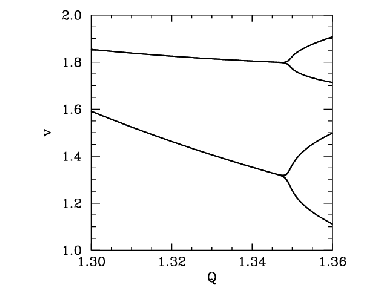Figure 76 is essentially a continuation of Fig 68. Data is shown for two sets of initial conditions which lead to motions converging on left-favouring (lower branch) and right-favouring (upper branch) periodic attractors. We have already seen that the left-favouring periodic attractor undergoes a period-doubling bifurcation at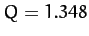. It is clear from Figure 76 that the right-favouring attractor undergoes a similar bifurcation at almost exactly the same-value. This is hardly surprising since, as has already been mentioned, for fixed physical parameters (i.e.,,,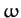), the left- and right-favouring attractors are mirror-images of one another.Next: Route to Chaos Up: The Chaotic Pendulum Previous: Basins of Attraction
Richard Fitzpatrick 2011-03-31